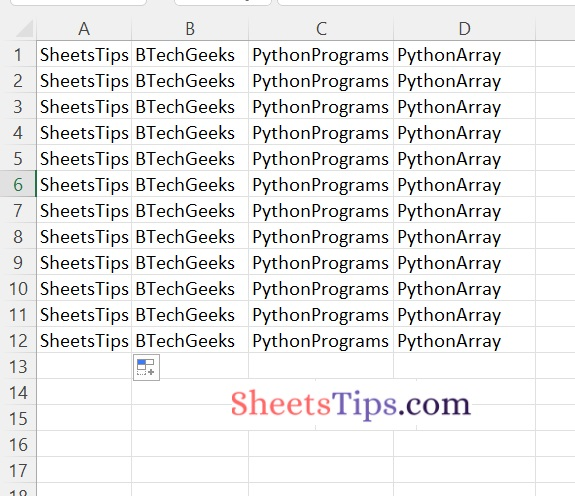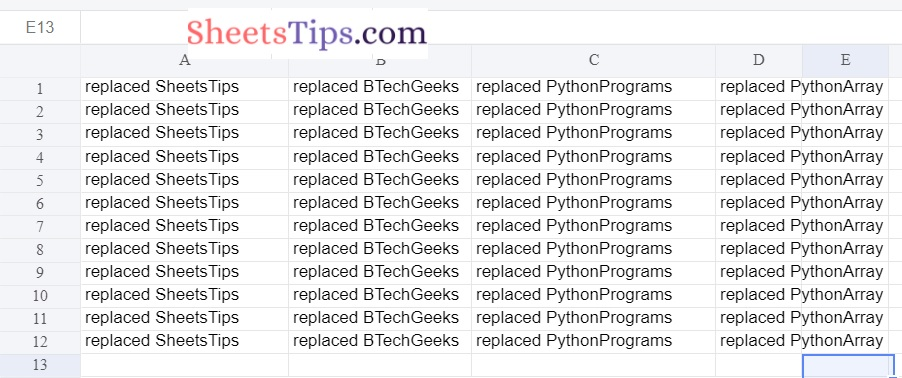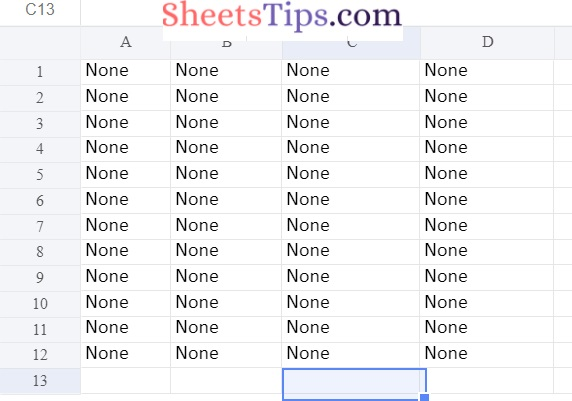# Python Program to Replace a Word in Excel

Excel is a very handy tool for displaying data in the form of rows and columns. We can state that before the database, excel played an important role in data storing. Many batch processes are now carried out using Excel input. The need to replace text in an Excel sheet is always present because Excel always contains crucial data. Let’s look at how to replace a word in Excel using Python in this article.

Excel File without replaced words:## Program to Replace a Word in Excel in Python

Below are the ways to replace a word in the excel File in Python:

### Method #1: Using xlwt & xlrd Packages

To install these packages, use the command below in the terminal.

1)xlwt

This package contains a pure Python module for creating spreadsheet files that are compatible with Excel 97/2000/XP/2003, OpenOffice.org Calc, and Gnumeric. Excel spreadsheets can be created on any platform that does not require Excel or a COM server.

Xlwt is a fork of the defunct pyExcelerator module that includes various bug fixes and upgrades. See the python-xlrd package for functionality previously offered by the parse xls function.

The Python 3.x module is included in this package.

Installation:

```# To write data into the excel File
pip install xlwt```

Output:

```Collecting xlwt
---------------------------------------- 100.0/100.0 KB 2.9 MB/s eta 0:00:00
Installing collected packages: xlwt
Successfully installed xlwt-1.3.0```

2)xlrd

To get data from a spreadsheet, use the xlrd module. Python, for example, can read, write, and modify data.

Furthermore, the user may be required to navigate across numerous sheets and extract data based on certain criteria, as well as alter some rows and columns and perform a great deal of labory. Use the xlrd module to extract data from a spreadsheet.

Before implementing the xlrd module, ensure that it is imported into the application using the command line listed below in CMD on your system.

Installation:

```# To read the data of the excel file
pip install xlrd```

Output:

```Collecting xlrd
---------------------------------------- 96.5/96.5 KB 5.8 MB/s eta 0:00:00
Installing collected packages: xlrd
Successfully installed xlrd-2.0.1```

The code below includes two Excel workbooks. One for reading and getting data. Other than that, for writing the replaced content. We can perform “n” replacements. The column should include the exact searched text that needs to be updated, and once found, it is replaced and written to a new workbook.

Approach:

• Import the xlwt module using the import keyword.
• Import the xlrd module using the import keyword.
• Read the excel file by providing the excel file path as an argument to the open_workbook() function of the xlrd module and store it in a variable.
• Get the sheet by passing the sheet name as an argument to the sheet_by_name() function and apply it to the workbook object
• Create a new WorkBook to store the replaced text using the Workbook() function of the xlwt module
• Create a new Sheet to store the replaced text using the add_sheet() function by passing the sheet Name as an argument to it and apply on the above created workBook Object.
• Store the replacement key value pairs in the dictionary.
• Iterate over the rows of the given sheet using the for loop.
• To get the number of rows of the sheet using nrows() attribute.
• Get the data value of each column of the sheet using the cell_value() function
• Iterate over the index and values of the dataValue using the for loop
• Check if the cell value present in replacementTextKeyValuePairs keys using keys() function
• If it is present then replace the cell value with the dictionary key value.
• Else replace with the original Value
• Save the replaced text work using the save() function.
• The Exit of the Program.

Below is the Implementation:

```# Import the xlwt module using the import keyword
import xlwt
# Import the xlrd module using the import keyword
import xlrd

# Read the excel file by providing the excel file path as an argument
# to the open_workbook() function of the xlrd module and store it in a variable
workbookObject = xlrd.open_workbook('replaceWordExcelFile.xlsx')
# Get the sheet by passing the sheet name as an argument to the sheet_by_name() function and apply it on the workbook object
sheetObject = workbookObject.sheet_by_name('Sheet1')

# Create a new WorkBook to store the replaced text using the Workbook() function of the xlwt module
workbookObjectForReplacementText = xlwt.Workbook()
# Create a new Sheet to store the replaced text using the add_sheet() function by passing
# the sheet Name as an agrument to it and apply on the above created workBook Object

# Store the replacement key value pairs as dictionary
replacementTextKeyValuePairs = {'SheetsTips': 'replaced SheetsTips',
'BTechGeeks': 'replaced BTechGeeks',
'PythonPrograms': 'replaced PythonPrograms',
'PythonArray':'replaced PythonArray'}
# Iterate over the rows of the given sheet using the for loop
# To get the number of rows of the sheet using nrows() attribute
for i in range(sheetObject.nrows):
print(i)

# Get the data value of each column of the sheet using the cell_value() function
dataValue = [sheetObject.cell_value(i, col)for col in range(sheetObject.ncols)]
# Iterate over the index and values of the dataValue using the for loop
for index, value in enumerate(dataValue):
# Check if the cell value present in replacementTextKeyValuePairs keys using keys() function
if value in replacementTextKeyValuePairs.keys():
# If it is present then replace the cell value with the dictionary key value
sheetObjectForReplacementText.write(
i, index, str(replacementTextKeyValuePairs.get(value)))
else:
# Else replace with  the original Value
sheetObjectForReplacementText.write(i, index, value)

# Save the replaced text work using the save() function
workbookObjectForReplacementText.save('excelFilewithreplacedtext.xlsx')
```

Output:

```0
1
2
3
4
5
6
7
8
9
10
11```

### Method #2: Using Openpyxl

Openpyxl Module:

OpenPyXL is a Python package that allows you to communicate with Excel (.xlxs) files. But you can’t do it with Pandas. Yes, however Pandas does not support charts, formulas, or images. OpenPyXL allows data scientists and analysts to conduct a wide range of operations on Excel files, including:

• Creating and renaming sheets.
• Drawing/Plotting charts.
• Creating pivot tables.
• Inserting images, and even doing formatting and styling.

Another noteworthy feature of OpenPyXL is that it does not require the installation of Microsoft Excel.

Installation:

`pip install openpyxl`

Output:

```Collecting openpyxl
---------------------------------------- 242.1/242.1 KB 14.5 MB/s eta 0:00:00
Requirement already satisfied: et-xmlfile in c:\users\cirus\appdata\local\programs\python\python310\lib\site-packages\et_xmlfile-1.1.0-py3.10.egg (from openpyxl) (1.1.0)
Installing collected packages: openpyxl
Successfully installed openpyxl-3.0.10```

Approach:

• We can do reading as well as writing into the excel file using a single openpyxl module
• Import the openpyxl module using the import Keyword
• Import the get_column_letter function from the openpyxl using import, from keywords
• Load / Read the excel file by giving the excel file path as an argument to the load_workbook() function of the openpyxl module and store this workbook object in a variable.
• Get all the sheet names of the workbook using the sheet names attribute and apply them on the workbook Object
• Get the worksheet of the workbook object and store it in a variable.
• Get the number of rows of the worksheet using the max_row attribute and store it in a variable.
• Get the number of columns of the worksheet using the max_column attribute and store it in a variable.
• Store the replacement key value pairs as dictionary.
• Iterate over the number of columns using the for loop
• Iterate till the number of rows using the nested for loop
• Get the cell value using the value attribute and by passing the row number and column number to the get_column_letter() function
• Traverse in the keys of the replacementTextKeyValuePairs dictionary using the for loop
• Check if the cell value is equal to the key using the If conditional Statement
• If it is equal then get the value of the key using the get() function and store it in a variable
• Assign the current cell with this new value
• Save the workbook by passing the path of the excel sheet using the save() function and apply to the above workbook object
• The Exit of the Program.

Below is the Implementation:

```# We can do reading as well as writing into the excel file using single openpyxl module
# Import the openpyxl module using the import Keyword
import openpyxl
# Import the get_column_letter function from the openpyxl using import , from keywords
from openpyxl.utils.cell import get_column_letter

# Load / Read the excel file by giving the excel file path as an argument to the load_workbook()
# function of the openpyxl module and store this workbook object in a variable.
# Get all the sheet names of the workbook using the sheetnames attribute and apply it on the workbook Object
print(workbookObject.sheetnames)
# Get the worksheet of the workbook object and store it in a variable
worksheetObject = workbookObject["Sheet1"]

# Get the number of rows of the worksheet using the max_row attribute and store it in a variable
numberOfRows = worksheet.max_row

# Get the number of columns of the worksheet using the max_column attribute and store it in a variable
numberOfColumns = worksheet.max_column

# Store the replacement key value pairs as dictionary
replacementTextKeyValuePairs = {'SheetsTips': 'replaced SheetsTips',
'BTechGeeks': 'replaced BTechGeeks',
'PythonPrograms': 'replaced PythonPrograms',
'PythonArray':'replaced PythonArray'}

# Iterate over the number of columns using the for loop
for i in range(numberOfColumns):
# Iterate till the number of rows using the nested for loop
for j in range(numberOfRows):
# Get the cell value using the value attribute and by passing the row number and column number to the get_column_letter() function
cell_Value = str(worksheetObject[get_column_letter(i+1)+str(j+1)].value)
# Traverse in the keys of the replacementTextKeyValuePairs dictionary using the for loop
for key in replacementTextKeyValuePairs.keys():
# Check if the cell value is equal to the key using the If conditional Statement
if str(cell_Value) == key:
# If it is equal then get value of the key using the get() function and store it in a variable
newCellValue = replacementTextKeyPairs.get(key)
# Assign the current cell with this new value
worksheetObject[get_column_letter(i+1)+str(j+1)] = str(newCellValue)
# Save the workbook by passing the path of excel sheet using the save() function and apply on the above workbook object
workbookObject.save('excelFilewithreplacedtext.xlsx')
```

Output:

```['Sheet1']```

### Method #3: Using Openpyxl to Replace with None Values

Below is the Implementation:

```# We can do reading as well as writing into the excel file using single openpyxl module
# Import the openpyxl module using the import Keyword
import openpyxl
# Import the get_column_letter function from the openpyxl using import , from keywords
from openpyxl.utils.cell import get_column_letter

# Load / Read the excel file by giving the excel file path as an argument to the load_workbook()
# function of the openpyxl module and store this workbook object in a variable.
# Get all the sheet names of the workbook using the sheetnames attribute and apply it on the workbook Object
print(workbookObject.sheetnames)
# Get the worksheet of the workbook object and store it in a variable
worksheetObject = workbookObject["Sheet1"]

# Get the number of rows of the worksheet using the max_row attribute and store it in a variable
numberOfRows = worksheet.max_row

# Get the number of columns of the worksheet using the max_column attribute and store it in a variable
numberOfColumns = worksheet.max_column

# Store the replacement key value pairs as dictionary
replacementTextKeyValuePairs = {'SheetsTips': None,
'BTechGeeks': None,
'PythonPrograms': None,
'PythonArray':None}

# Iterate over the number of columns using the for loop
for i in range(numberOfColumns):
# Iterate till the number of rows using the nested for loop
for j in range(numberOfRows):
# Get the cell value using the value attribute and by passing the row number and column number to the get_column_letter() function
cell_Value = str(worksheetObject[get_column_letter(i+1)+str(j+1)].value)
# Traverse in the keys of the replacementTextKeyValuePairs dictionary using the for loop
for key in replacementTextKeyValuePairs.keys():
# Check if the cell value is equal to the key using the If conditional Statement
if str(cell_Value) == key:
# If it is equal then get value of the key using the get() function and store it in a variable
newCellValue = replacementTextKeyPairs.get(key)
# Assign the current cell with this new value
worksheetObject[get_column_letter(i+1)+str(j+1)] = str(newCellValue)
# Save the workbook by passing the path of excel sheet using the save() function and apply on the above workbook object
workbookObject.save('excelFilewithreplacedtext.xlsx')
```

Output:

```None	None	None	None
None	None	None	None
None	None	None	None
None	None	None	None
None	None	None	None
None	None	None	None
None	None	None	None
None	None	None	None
None	None	None	None
None	None	None	None
None	None	None	None
None	None	None	None
```